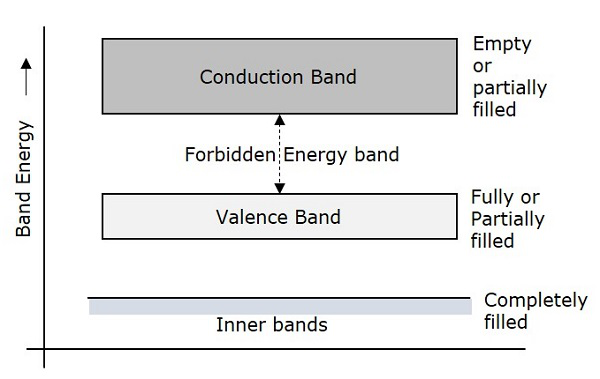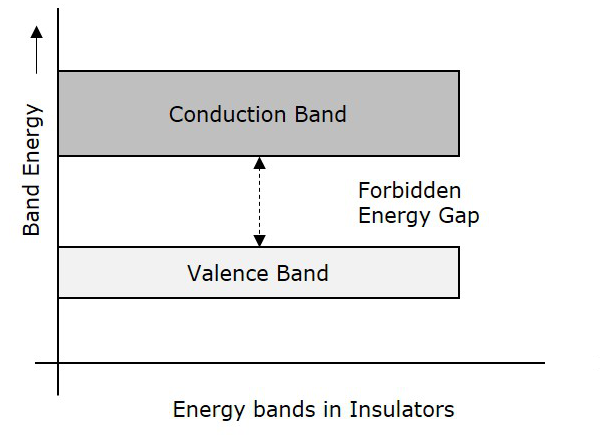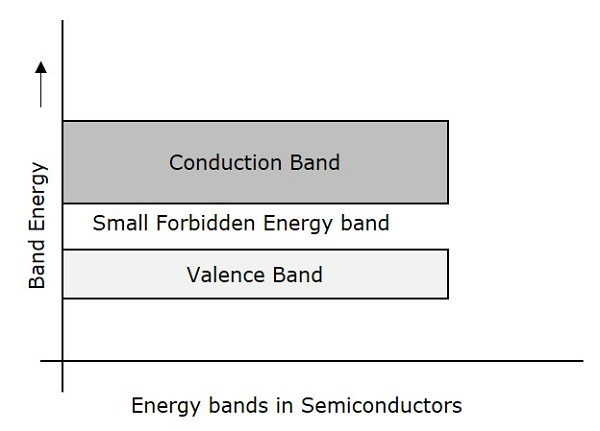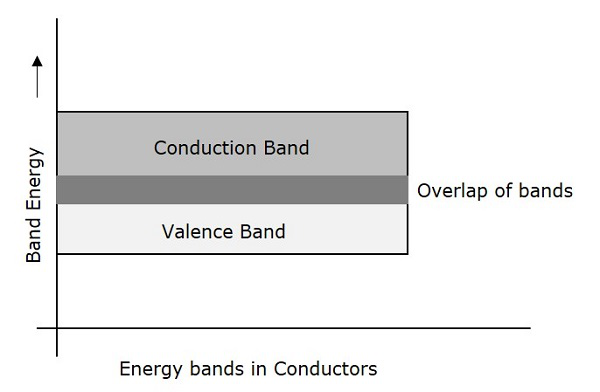# Basic Electronics – Energy Bands

In gaseous substances, the arrangement of molecules is not close. In liquids, the molecular arrangement is moderate. But, in solids, the molecules are so closely arranged, that the electrons in the atoms of molecules tend to move into the orbitals of neighboring atoms. Hence the electron orbitals overlap when the atoms come together.

Due to the intermixing of atoms in solids, instead of single energy levels, there will be bands of energy levels formed. These set of energy levels, which are closely packed are called as Energy bands.

## Valance Band

The electrons move in the atoms in certain energy levels but the energy of the electrons in the innermost shell is higher than the outermost shell electrons. The electrons that are present in the outermost shell are called as Valance Electrons.

These valance electrons, containing a series of energy levels, form an energy band which is called as Valence Band. The valence band is the band having the highest occupied energy.

## Conduction Band

The valence electrons are so loosely attached to the nucleus that even at room temperature, few of the valence electrons leave the band to be free. These are called as free electrons as they tend to move towards the neighboring atoms.

These free electrons are the ones which conduct the current in a conductor and hence called as Conduction Electrons. The band which contains conduction electrons is called as Conduction Band. The conduction band is the band having the lowest occupied energy.

## Forbidden gap

The gap between valence band and conduction band is called as forbidden energy gap. As the name implies, this band is the forbidden one without energy. Hence no electron stays in this band. The valence electrons, while going to the conduction band, pass through this.

The forbidden energy gap if greater, means that the valence band electrons are tightly bound to the nucleus. Now, in order to push the electrons out of the valence band, some external energy is required, which would be equal to the forbidden energy gap.

The following figure shows the valance band, conduction band, and the forbidden gap.Depending upon the size of the forbidden gap, the Insulators, the Semiconductors and the Conductors are formed.

## Insulators

Insulators are such materials in which the conduction cannot take place, due to the large forbidden gap. Examples: Wood, Rubber. The structure of energy bands in Insulators is as shown in the following figure.### Characteristics

The following are the characteristics of Insulators.

• The Forbidden energy gap is very large.

• Valance band electrons are bound tightly to atoms.

• The value of forbidden energy gap for an insulator will be of 10eV.

• For some insulators, as the temperature increases, they might show some conduction.

• The resistivity of an insulator will be in the order of 107 ohm-meter.

## Semiconductors

Semiconductors are such materials in which the forbidden energy gap is small and the conduction takes place if some external energy is applied. Examples: Silicon, Germanium. The following figure shows the structure of energy bands in semiconductors.### Characteristics

The following are the characteristics of Semiconductors.

• The Forbidden energy gap is very small.

• The forbidden gap for Ge is 0.7eV whereas for Si is 1.1eV.

• A Semiconductor actually is neither an insulator, nor a good conductor.

• As the temperature increases, the conductivity of a semiconductor increases.

• The conductivity of a semiconductor will be in the order of 102 mho-meter.

## Conductors

Conductors are such materials in which the forbidden energy gap disappears as the valence band and conduction band become very close that they overlap. Examples: Copper, Aluminum. The following figure shows the structure of energy bands in conductors.### Characteristics

The following are the characteristics of Conductors.

• There exists no forbidden gap in a conductor.

• The valance band and the conduction band gets overlapped.

• The free electrons available for conduction are plenty.

• A slight increase in voltage, increases the conduction.

• There is no concept of hole formation, as a continuous flow of electrons contribute the current.

## Important Terms

There is a necessity to discuss a few important terms here before we move on to subsequent chapters.

### Current

It is simply the flow of electrons. A continuous flow of electrons or charged particles, can be termed as Current. It is indicated by I or i. It is measured in Amperes. This can be alternating current AC or direct current DC.

### Voltage

It is the potential difference. When there occurs a difference in potentialities, between two points, there is said to be a voltage difference, measured between those two points. It is indicated by V. It is measured in Volts.

### Resistance

It is the property of opposing the flow of electrons. The possession of this property can be termed as resistivity. This will be discussed later in detail.

## Ohms Law

With the terms discussed above, we have a standard law, which is very crucial for the behavior of all the electronic components, called as Ohms Law. This states the relation between current and voltage in an ideal conductor.

According to Ohms law, the potential difference across an ideal conductor is proportional to the current through it.

$$V\:\alpha\:\:I$$

An ideal conductor has no resistance. But in practice, every conductor has some resistance in it. As the resistance increases, the potential drop also increases and hence the voltage increases.

Hence the voltage is directly proportional to the resistance it offers.

$$V\:\alpha\:\:R$$

$$V = IR$$

But the current is inversely proportional to the resistance.

$$V\:\alpha\:\:I\:\alpha\:\:\frac{1}{R}$$

$$I = V/R$$

Hence, in practice, an Ohms law can be stated as –

According to Ohm`s law, the current flowing through a conductor is proportional to the potential difference across it, and is inversely proportional to the resistance it offers.

This law is helpful in determining the values of unknown parameters among the three which help to analyze a circuit.